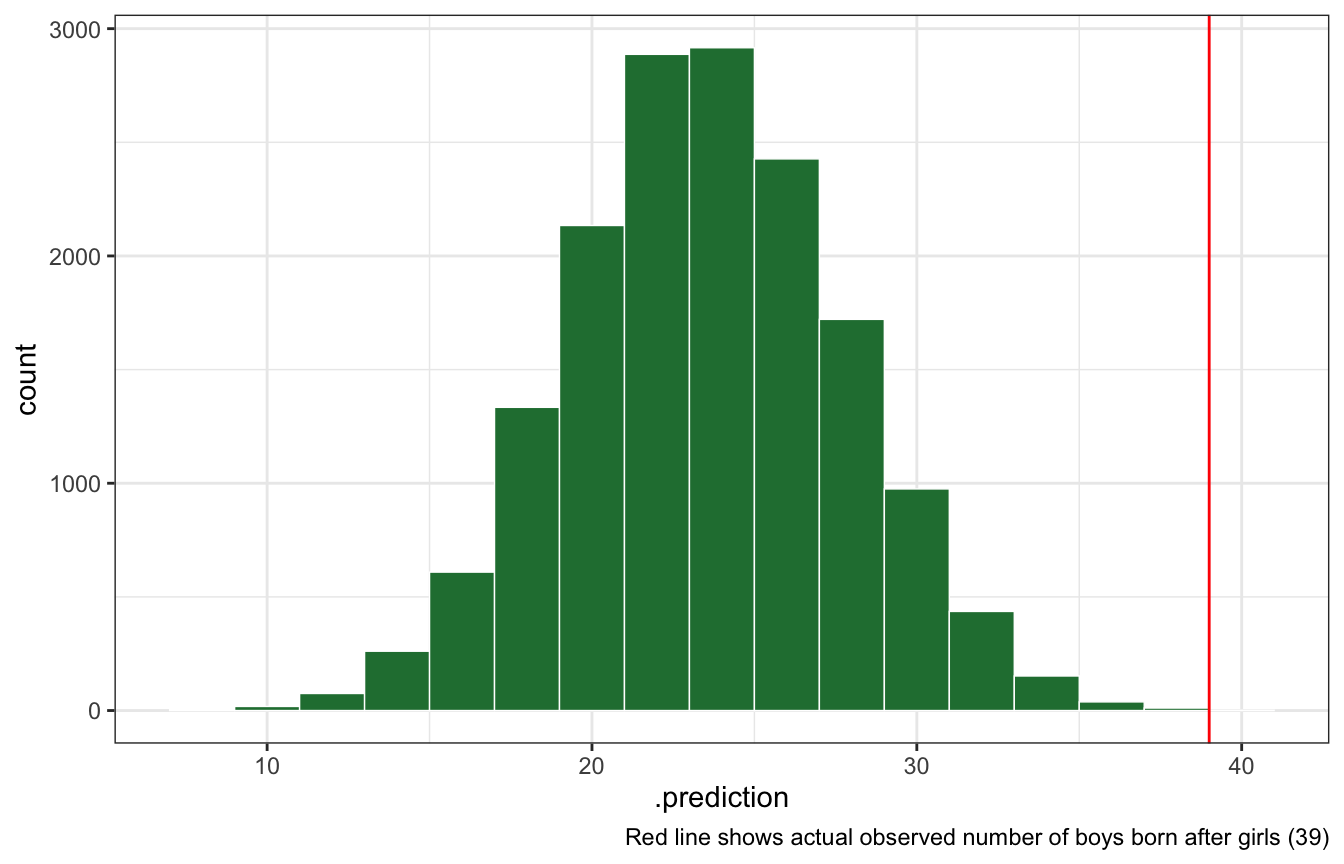Posteriors from grids

Published

September 2, 2022

``````library(tidyverse)
library(brms)
library(tidybayes)
library(ggdist)
library(broom.mixed)
library(glue)

# Plot stuff
clrs <- MetBrewer::met.brewer("Lakota", 6)
theme_set(theme_bw())

# Seed stuff
BAYES_SEED <- 1234
set.seed(1234)``````

This `births` data shows the sex of the first and second children born to 100 different families (`1` = boy, `2` = girl). The first family had a boy then a girl, the second had a girl and then a boy, the thrid had no boys, etc.

``````data(homeworkch3, package = "rethinking")

all_births <- tibble(birth1, birth2)
all_births
## # A tibble: 100 × 2
##    birth1 birth2
##     <dbl>  <dbl>
##  1      1      0
##  2      0      1
##  3      0      0
##  4      0      1
##  5      1      0
##  6      1      1
##  7      0      1
##  8      1      1
##  9      0      0
## 10      1      0
## # … with 90 more rows``````

In these exercises we don’t care about birth order, so we’ll pool all the births into one long 200-birth vector:

``````births <- all_births %>%
pivot_longer(cols = everything(), values_to = "boy")
births
## # A tibble: 200 × 2
##    name     boy
##    <chr>  <dbl>
##  1 birth1     1
##  2 birth2     0
##  3 birth1     0
##  4 birth2     1
##  5 birth1     0
##  6 birth2     0
##  7 birth1     0
##  8 birth2     1
##  9 birth1     1
## 10 birth2     0
## # … with 190 more rows``````

What proportion of births were boys?

``````births_summary <- births %>%
summarize(n_boys = sum(boy),
prop_boys = n_boys / n())
births_summary
## # A tibble: 1 × 2
##   n_boys prop_boys
##    <dbl>     <dbl>
## 1    111     0.555

n_boys <- pull(births_summary, n_boys)
prop_boys <- pull(births_summary, prop_boys)
total_births <- nrow(births)
n_girls <- total_births - n_boys``````

## 3H1

Using grid approximation, compute the posterior distribution for the probability of being a boy. Assume a uniform prior probability. Which parameter value maximizes the posterior probability?

``````birth_grid <- tibble(p_grid = seq(0, 1, length.out = 1001),
prior_uniform = 1) %>%
mutate(likelihood = dbinom(n_boys, size = total_births, prob = p_grid)) %>%
mutate(posterior_uniform = (likelihood * prior_uniform) / sum(likelihood * prior_uniform))
birth_grid
## # A tibble: 1,001 × 4
##    p_grid prior_uniform likelihood posterior_uniform
##     <dbl>         <dbl>      <dbl>             <dbl>
##  1  0                 1  0                 0
##  2  0.001             1  2.48e-275         4.98e-276
##  3  0.002             1  5.89e-242         1.18e-242
##  4  0.003             1  1.89e-222         3.81e-223
##  5  0.004             1  1.28e-208         2.57e-209
##  6  0.005             1  6.68e-198         1.34e-198
##  7  0.006             1  3.76e-189         7.56e-190
##  8  0.007             1  9.28e-182         1.86e-182
##  9  0.008             1  2.32e-175         4.66e-176
## 10  0.009             1  1.01e-169         2.03e-170
## # … with 991 more rows``````
``````birth_grid %>%
ggplot(aes(x = p_grid, y = posterior_uniform)) +
geom_area(fill = clrs)``````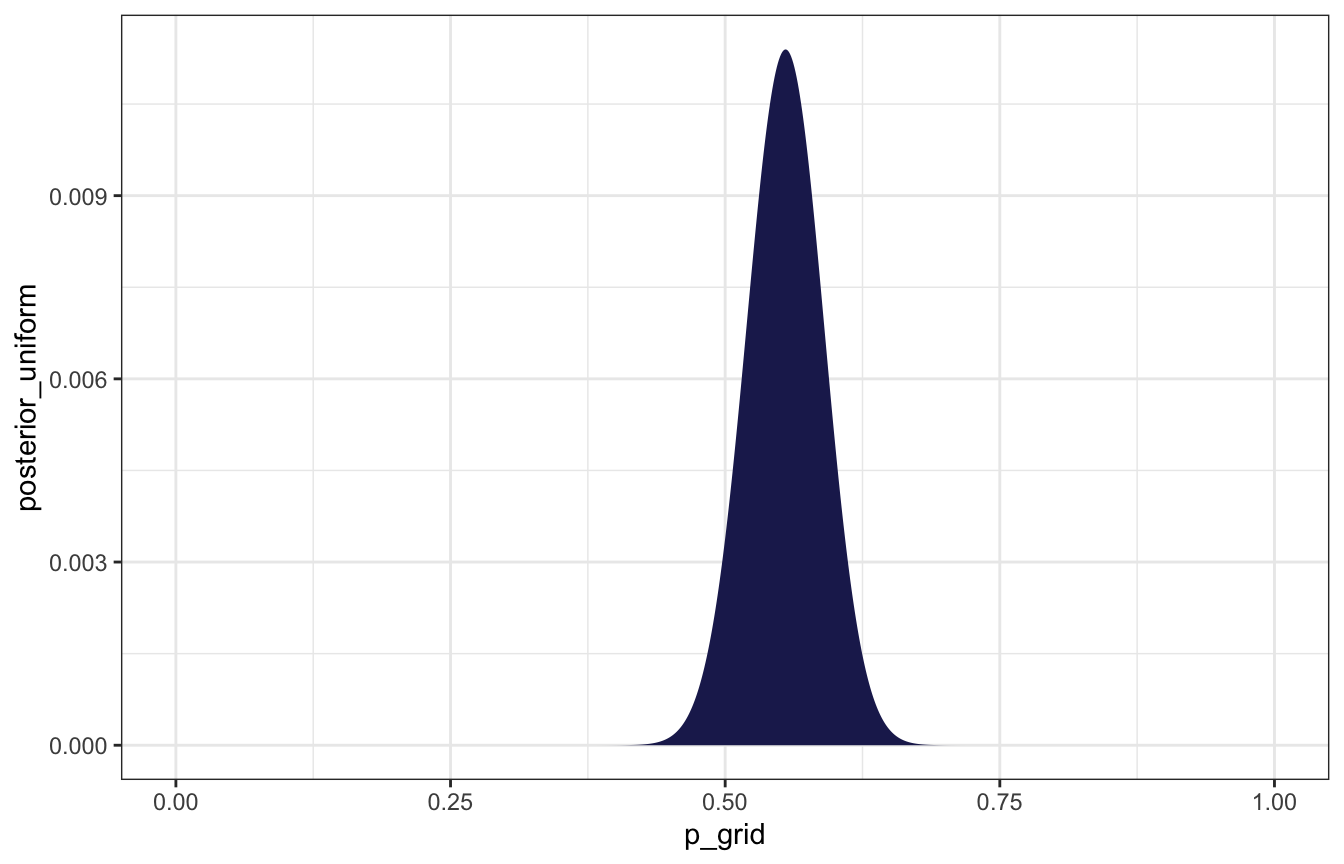Parameter that maximizes the probability:

``````birth_grid %>%
filter(posterior_uniform == max(posterior_uniform))
## # A tibble: 1 × 4
##   p_grid prior_uniform likelihood posterior_uniform
##    <dbl>         <dbl>      <dbl>             <dbl>
## 1  0.555             1     0.0567            0.0114``````

### With brms

``````model_births <- brm(
bf(boy | trials(total_births) ~ 0 + Intercept),
data = list(boy = n_boys, total_births = total_births),
prior = prior(beta(1, 1), class = b, lb = 0, ub = 1),
iter = 5000, warmup = 1000, seed = BAYES_SEED, cores = 4
)
## Compiling Stan program...
## Trying to compile a simple C file
## Start sampling``````
``````model_births %>%
gather_draws(b_Intercept) %>%
ggplot(aes(x = .value, y = .variable)) +
stat_halfeye(fill = clrs)``````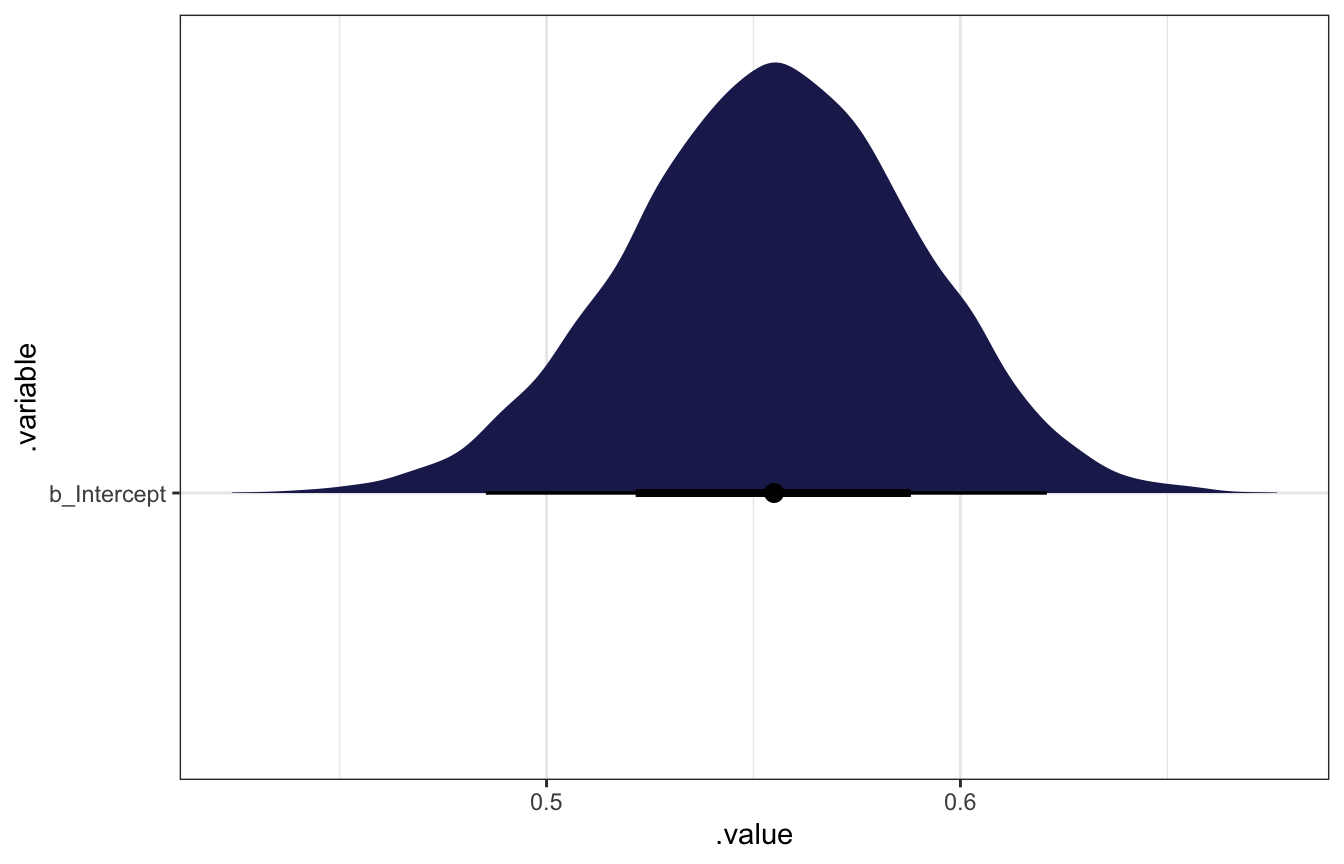``````model_births %>%
gather_draws(b_Intercept) %>%
summarize(median = median(.value))
## # A tibble: 1 × 2
##   .variable   median
##   <chr>        <dbl>
## 1 b_Intercept  0.555``````

## 3H2

Using the `sample` function, draw 10,000 random parameter values from the posterior distribution you calculated above. Use these samples to estimate the 50%, 89%, and 97% highest posterior density intervals.

``````birth_samples <- sample(birth_grid\$p_grid, prob = birth_grid\$posterior_uniform,
size = 10000, replace = TRUE)

tibble(x = birth_samples) %>%
ggplot(aes(x = x)) +
stat_slab(
aes(fill_ramp = stat(level)),
.width = c(0.02, 0.5, 0.89, 0.97, 1),
fill = clrs
) +
scale_fill_ramp_discrete(range = c(0.2, 1), guide = "none")``````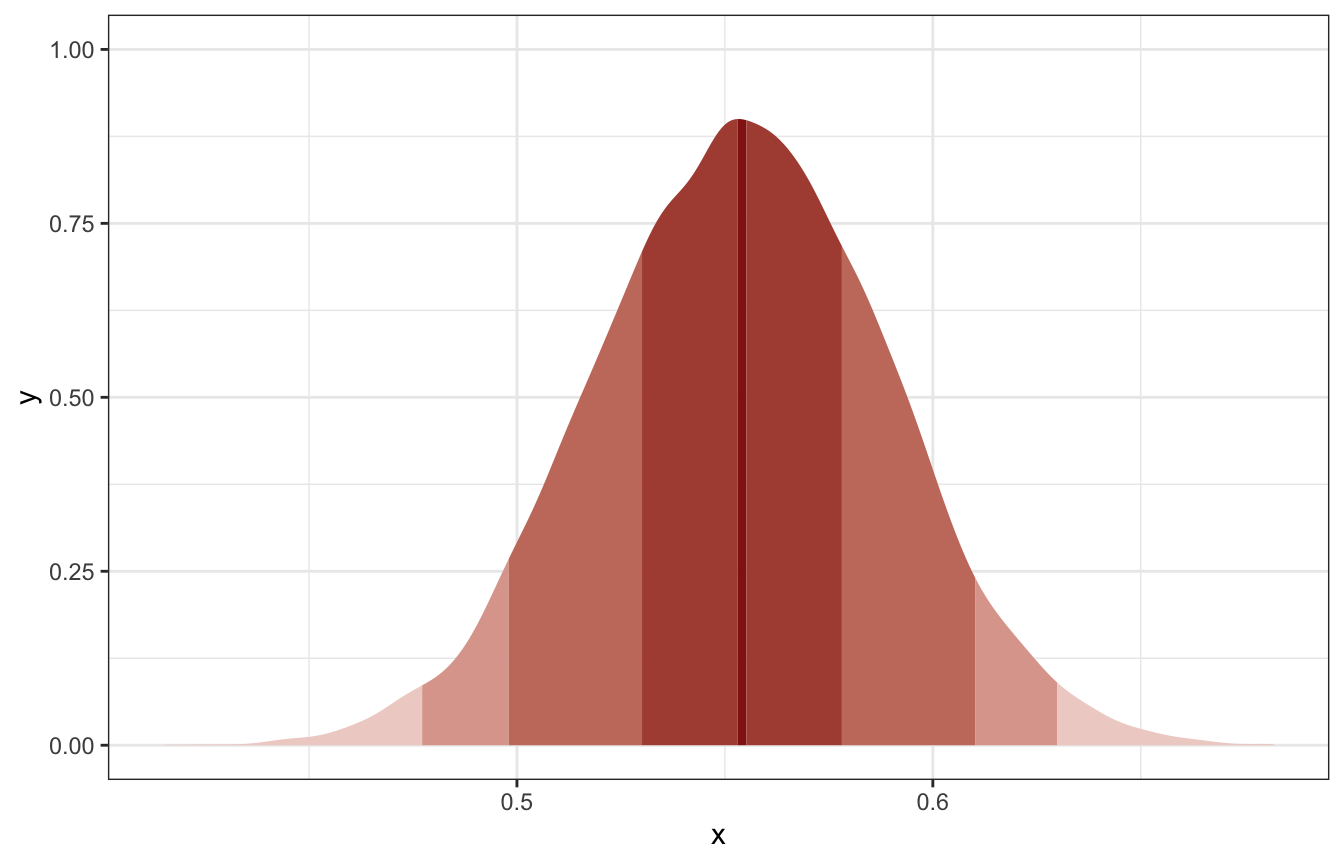``````
HDInterval::hdi(birth_samples, credMass = 0.5)
## lower upper
## 0.530 0.577
## attr(,"credMass")
##  0.5
HDInterval::hdi(birth_samples, credMass = 0.89)
## lower upper
## 0.494 0.605
## attr(,"credMass")
##  0.89
HDInterval::hdi(birth_samples, credMass = 0.97)
## lower upper
## 0.473 0.626
## attr(,"credMass")
##  0.97``````

### With brms

``````model_births %>%
median_hdci(b_Intercept, .width = c(0.5, 0.89, 0.97))
## # A tibble: 3 × 6
##   b_Intercept .lower .upper .width .point .interval
##         <dbl>  <dbl>  <dbl>  <dbl> <chr>  <chr>
## 1       0.555  0.532  0.578   0.5  median hdci
## 2       0.555  0.501  0.612   0.89 median hdci
## 3       0.555  0.480  0.629   0.97 median hdci``````
``````model_births %>%
tidy_draws() %>%
ggplot(aes(x = b_Intercept)) +
stat_slab(
aes(fill_ramp = stat(level)),
.width = c(0.02, 0.5, 0.89, 0.97, 1),
fill = clrs
) +
scale_fill_ramp_discrete(range = c(0.2, 1), guide = "none")``````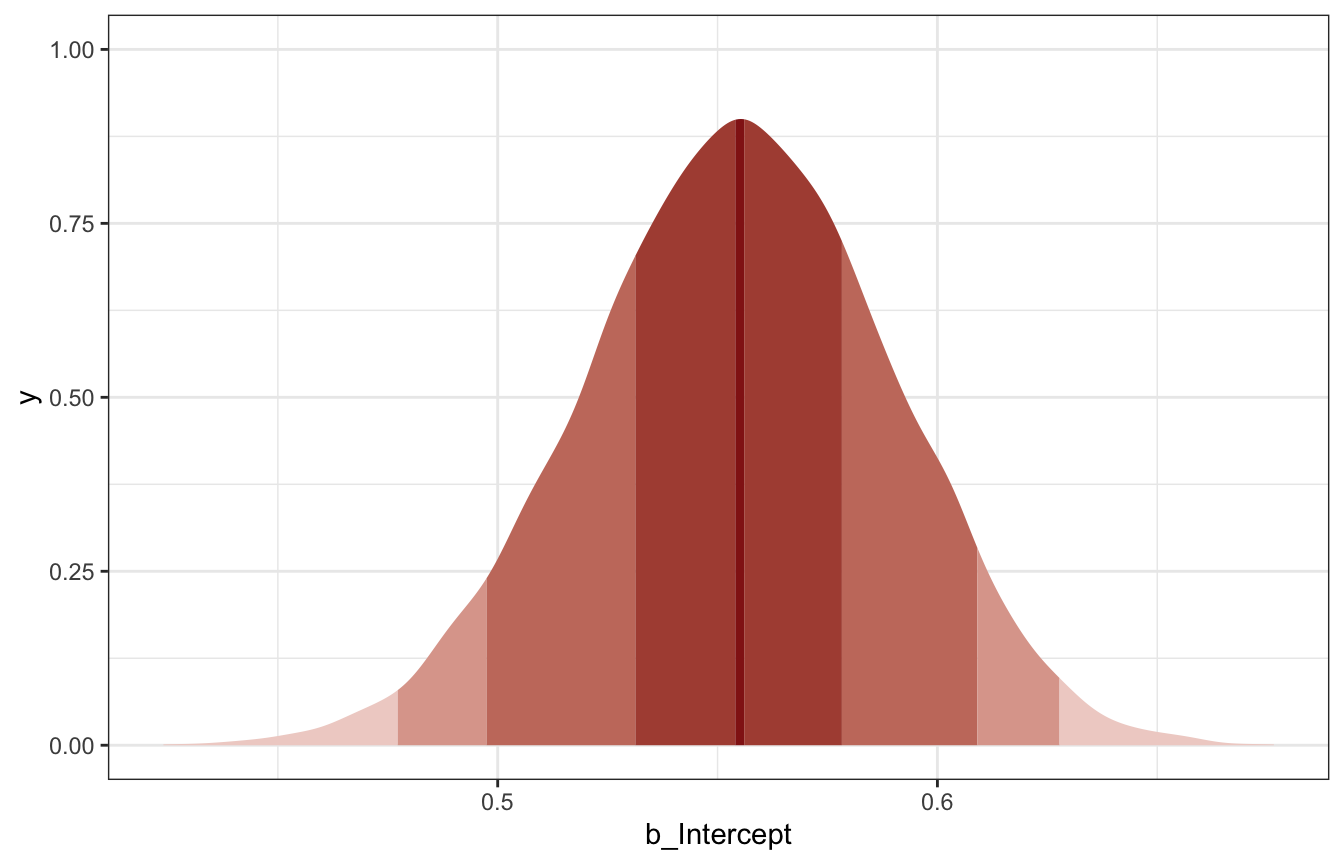## 3H3

Use `rbinom` to simulate 10,000 replicates of 200 births. You should end up with 10,000 numbers, each one a acount of boyts out of 200 births. Compare the distribution of predicted numbers of boys to the actual count in the data (111 boys out of 200 births). … Does it look like the model fits the data well? That is, does the distribution of predcitions include the actual observation as a central, likely outcome?

Looks good!

``````# Posterior predictive distribution
posterior_pred_births <- rbinom(10000, size = 200, prob = birth_samples)

posterior_pred_births %>%
enframe() %>%
ggplot(aes(x = value)) +
geom_histogram(binwidth = 2, color = "white", size = 0.25, fill = clrs) +
geom_vline(xintercept = n_boys, color = "red") +
labs(caption = glue("Red line shows actual observed number of boys ({n_boys})"))``````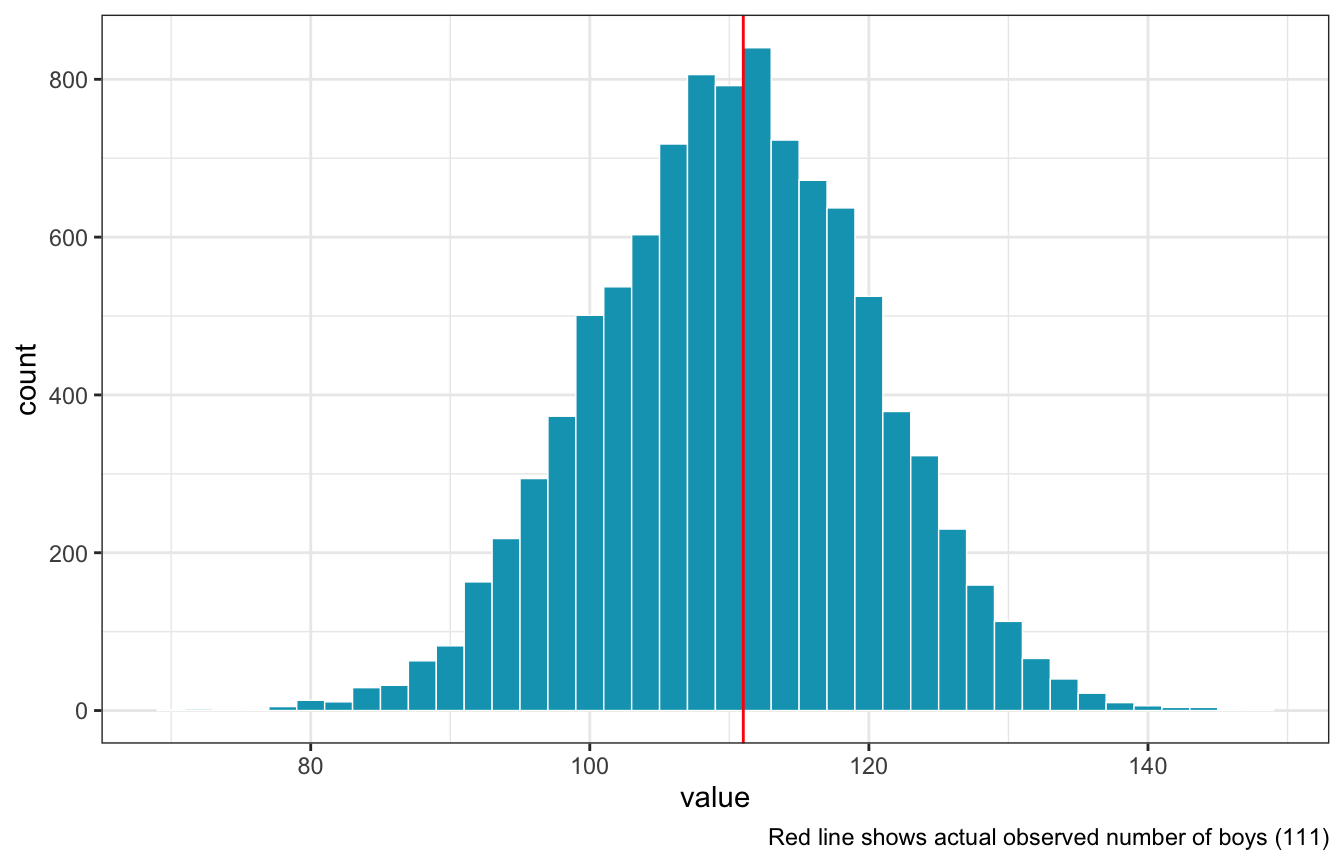### With brms

``````model_births %>%
predicted_draws(newdata = tibble(total_births = 200)) %>%
ggplot(aes(x = .prediction)) +
geom_histogram(binwidth = 2, color = "white", size = 0.25, fill = clrs) +
geom_vline(xintercept = n_boys, color = "red") +
labs(caption = glue("Red line shows actual observed number of boys ({n_boys})"))``````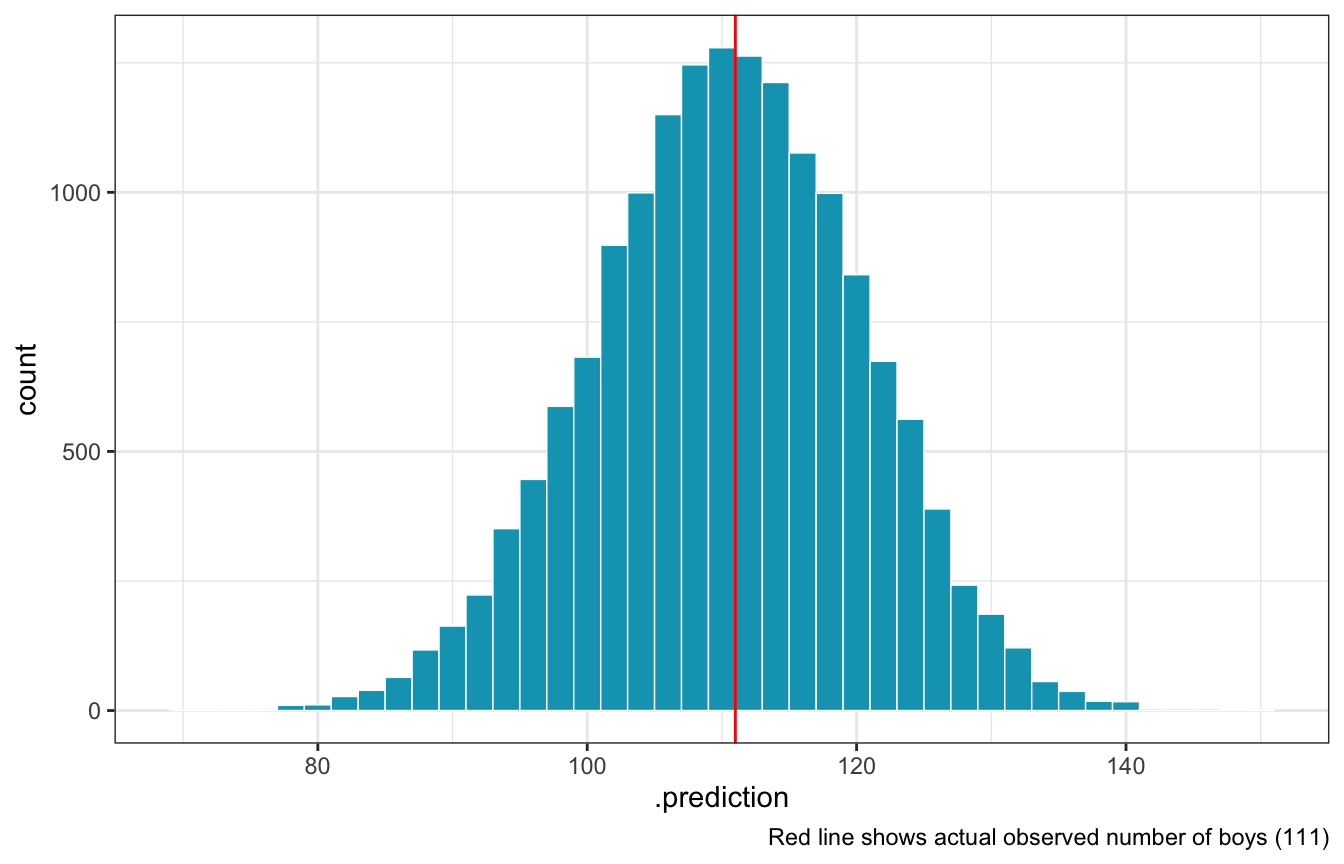## 3H4

Now compare 10,000 counts of boys from 100 simulated first borns only to the number of boys in the first births, `birth1`. How does the model look in this light?

We need to just look at first births:

``````n_boys_first <- sum(birth1)
total_births_first <- length(birth1)

birth_grid_first <- tibble(p_grid = seq(0, 1, length.out = 1001),
prior_uniform = 1) %>%
mutate(likelihood = dbinom(n_boys_first, size = total_births_first, prob = p_grid)) %>%
mutate(posterior_uniform = (likelihood * prior_uniform) / sum(likelihood * prior_uniform))

first_samples <- sample(birth_grid_first\$p_grid, prob = birth_grid_first\$posterior_uniform,
size = 10000, replace = TRUE)

posterior_pred_first <- rbinom(10000, size = 100, prob = first_samples)

posterior_pred_first %>%
enframe() %>%
ggplot(aes(x = value)) +
geom_histogram(binwidth = 2, color = "white", size = 0.25, fill = clrs) +
geom_vline(xintercept = n_boys_first, color = "red") +
labs(caption = glue("Red line shows actual observed number of firstborn boys ({n_boys_first})"))``````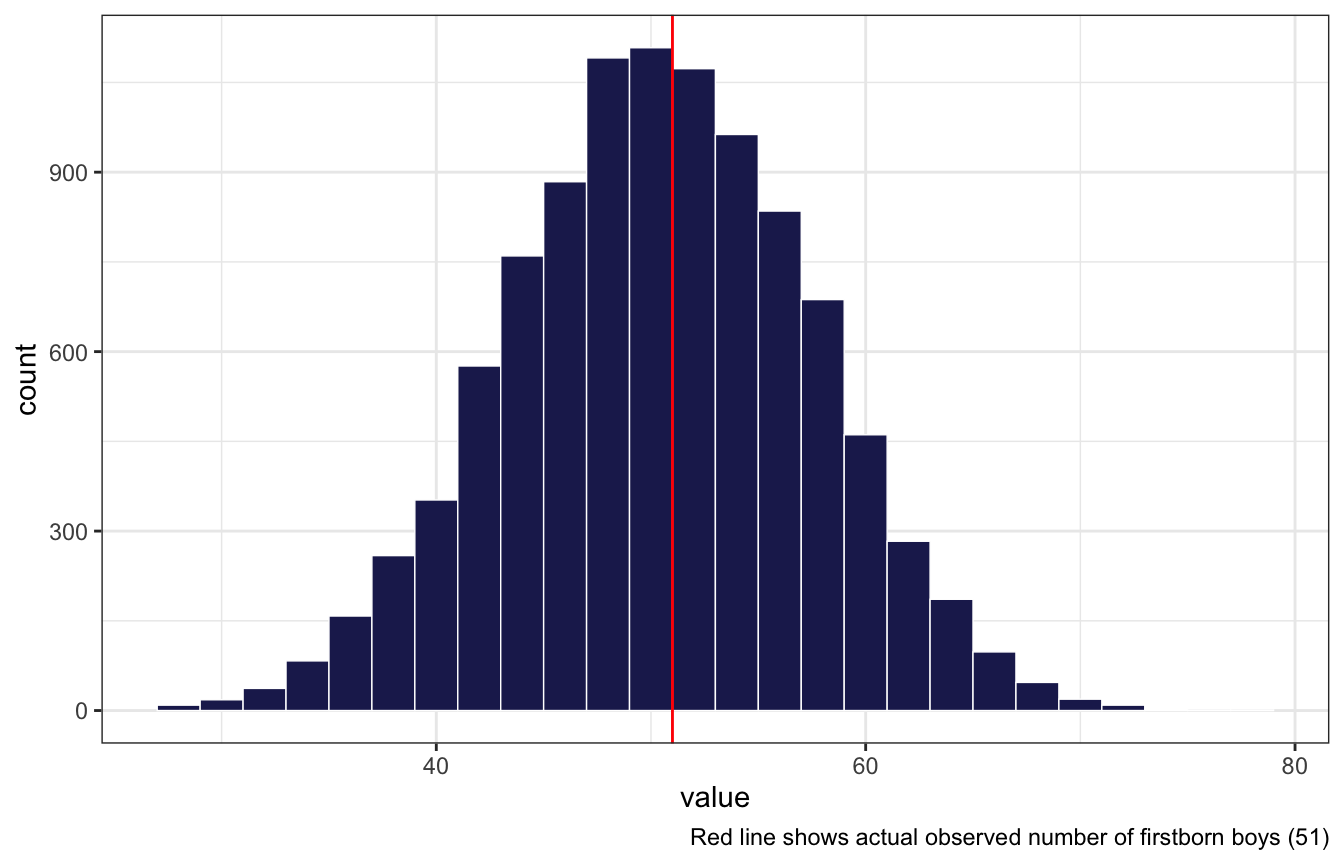Looks good still!

``````model_births_first <- brm(
bf(boy | trials(total_births) ~ 0 + Intercept),
data = list(boy = n_boys_first, total_births = total_births_first),
prior = prior(beta(1, 1), class = b, lb = 0, ub = 1),
iter = 5000, warmup = 1000, seed = BAYES_SEED, cores = 4
)
## Compiling Stan program...
## recompiling to avoid crashing R session
## Trying to compile a simple C file
## Start sampling``````

### With brms

Looks the same with brms too:

``````model_births_first %>%
predicted_draws(newdata = tibble(total_births = 100)) %>%
ggplot(aes(x = .prediction)) +
geom_histogram(binwidth = 2, color = "white", size = 0.25, fill = clrs) +
geom_vline(xintercept = n_boys_first, color = "red") +
labs(caption = glue("Red line shows actual observed number of firstborn boys ({n_boys_first})"))``````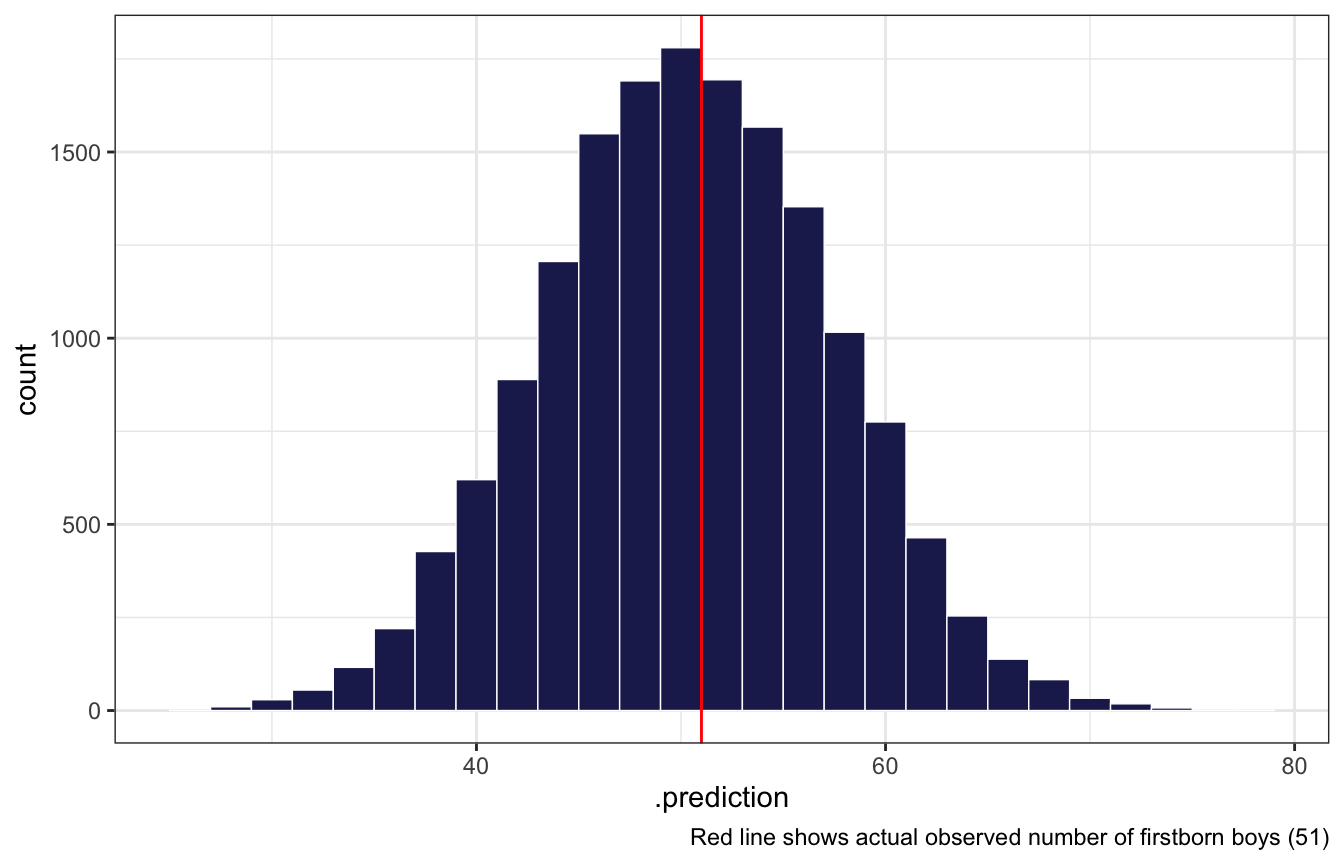## 3H5

The model assumes that sex of first and second births are independent. To check this assumption, focus now on second births that followed female first borns. Compare 10,000 simulated conts of boys to only those second births that followed girls. To do this correctly, you need to count the number of first borns who were girls and simulate that many births, 10,000 times. Compare the counts of boys in your simulations to the actual observed count of boys following girls. How does the model look in this light? Any guesses what is going on in these data?

``````n_girls_first <- length(birth1) - sum(birth1)
n_boys_after_girls <- all_births %>%
filter(birth1 == 0) %>%  # All families with a firstborn girl
summarize(boy_after_girl = sum(birth2)) %>%
pull(boy_after_girl)

posterior_pred_first_girl <- rbinom(10000, size = n_girls_first, prob = first_samples)

posterior_pred_first_girl %>%
enframe() %>%
ggplot(aes(x = value)) +
geom_histogram(binwidth = 2, color = "white", size = 0.25, fill = clrs) +
geom_vline(xintercept = n_boys_after_girls, color = "red") +
labs(caption = glue("Red line shows actual observed number of boys born after girls ({n_boys_after_girls})"))``````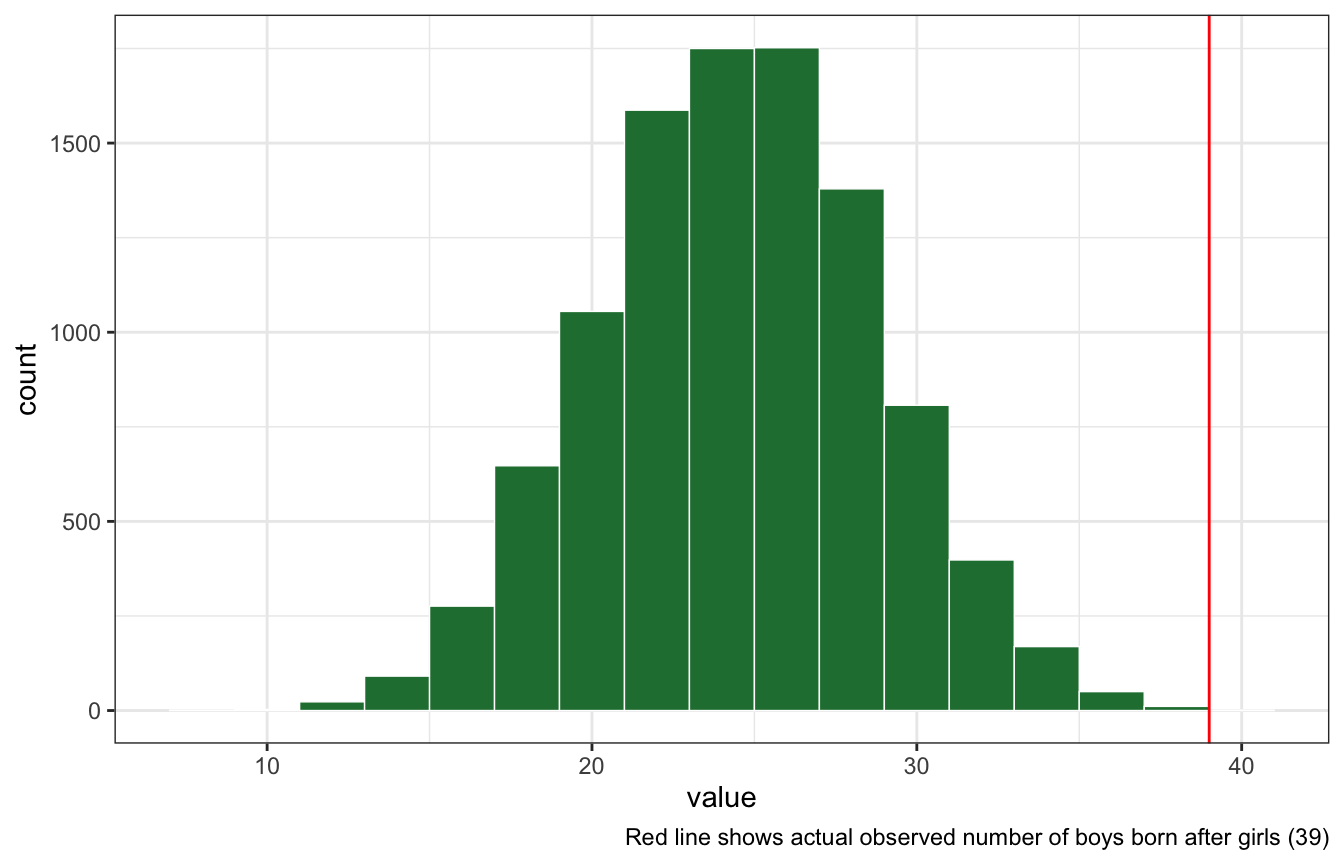### With brms

``````model_births_first_girls <- brm(
bf(girl | trials(total_births) ~ 0 + Intercept),
data = list(girl = n_girls_first, total_births = total_births_first),
prior = prior(beta(1, 1), class = b, lb = 0, ub = 1),
iter = 5000, warmup = 1000, seed = BAYES_SEED, cores = 4
)
## Compiling Stan program...
## recompiling to avoid crashing R session
## Trying to compile a simple C file
## Start sampling``````
``````model_births_first_girls %>%
predicted_draws(newdata = tibble(total_births = n_girls_first)) %>%
ggplot(aes(x = .prediction)) +
geom_histogram(binwidth = 2, color = "white", size = 0.25, fill = clrs) +
geom_vline(xintercept = n_boys_after_girls, color = "red") +
labs(caption = glue("Red line shows actual observed number of boys born after girls ({n_boys_after_girls})"))``````